Скачать презентацию Topic 10 — Linear Regression Least squares

624255210d09d259bc4e0072fccbc05c.ppt

• Количество слайдов: 28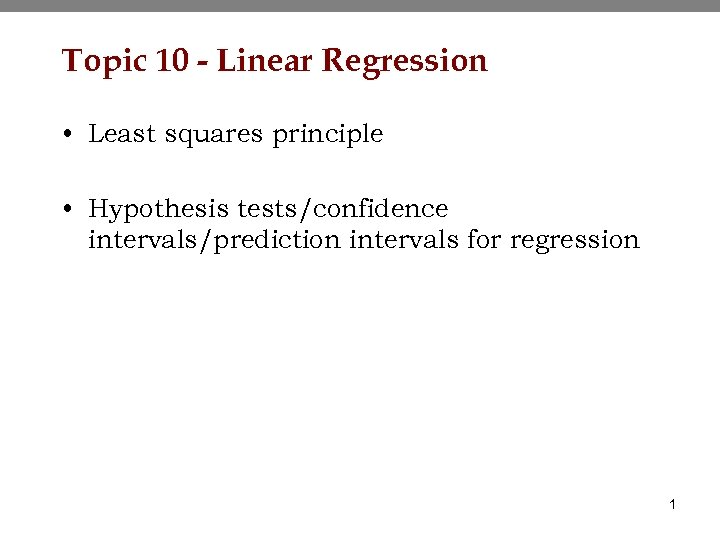Topic 10 - Linear Regression • Least squares principle • Hypothesis tests/confidence intervals/prediction intervals for regression 1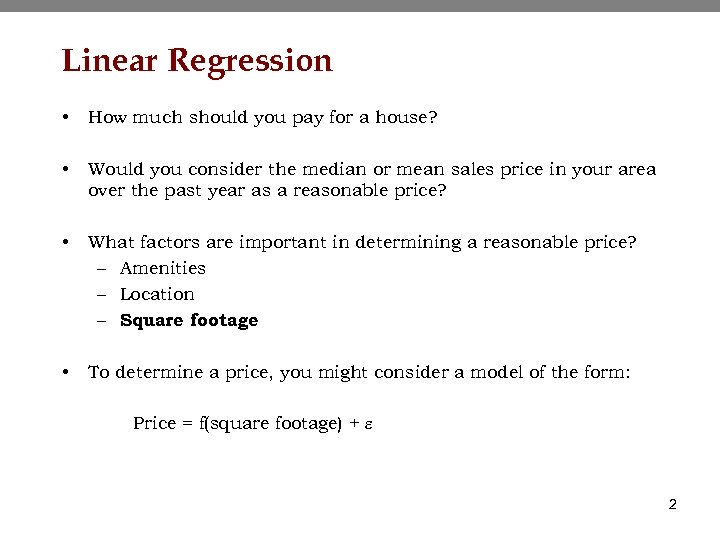Linear Regression • How much should you pay for a house? • Would you consider the median or mean sales price in your area over the past year as a reasonable price? • What factors are important in determining a reasonable price? – Amenities – Location – Square footage • To determine a price, you might consider a model of the form: Price = f(square footage) + e 2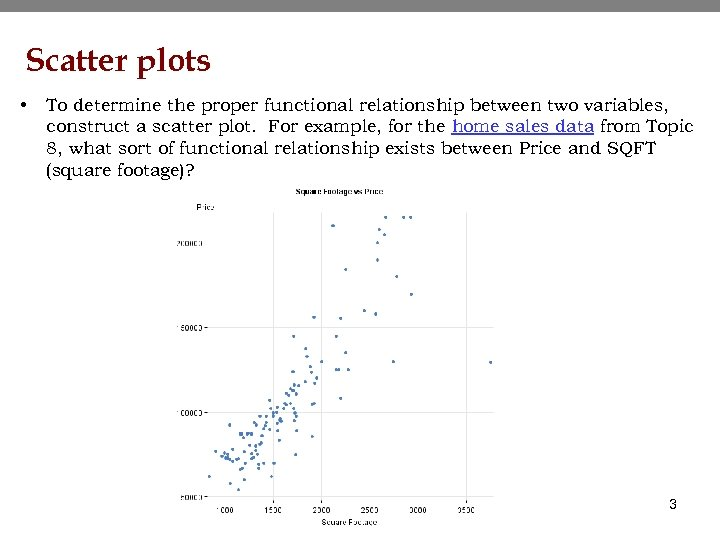Scatter plots • To determine the proper functional relationship between two variables, construct a scatter plot. For example, for the home sales data from Topic 8, what sort of functional relationship exists between Price and SQFT (square footage)? 3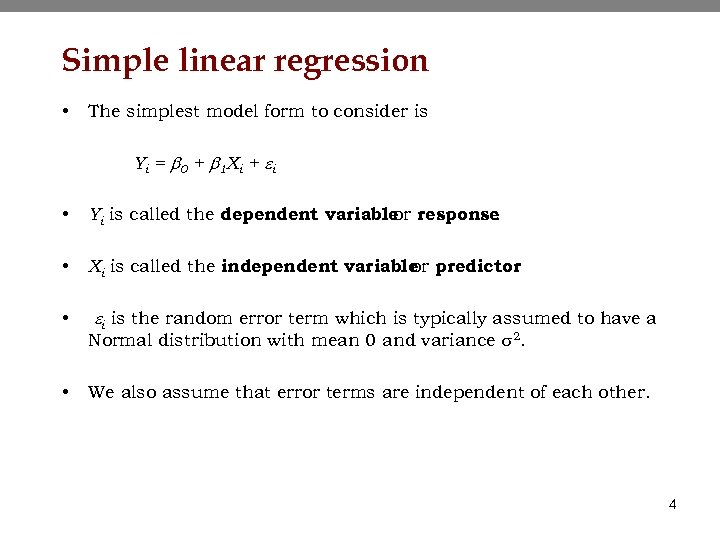Simple linear regression • The simplest model form to consider is Yi = b 0 + b 1 Xi + e i • Yi is called the dependent variable response or. • Xi is called the independent variable predictor or. • ei is the random error term which is typically assumed to have a • Normal distribution with mean 0 and variance s 2. We also assume that error terms are independent of each other. 4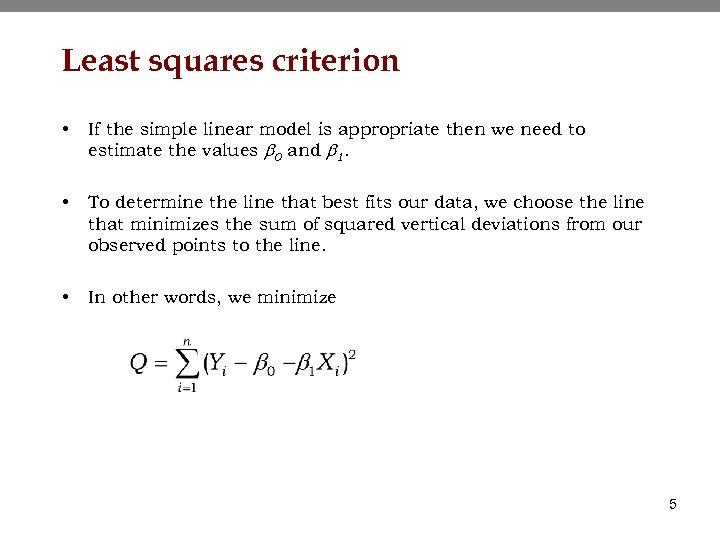Least squares criterion • If the simple linear model is appropriate then we need to estimate the values b 0 and b 1. • To determine the line that best fits our data, we choose the line that minimizes the sum of squared vertical deviations from our observed points to the line. • In other words, we minimize 5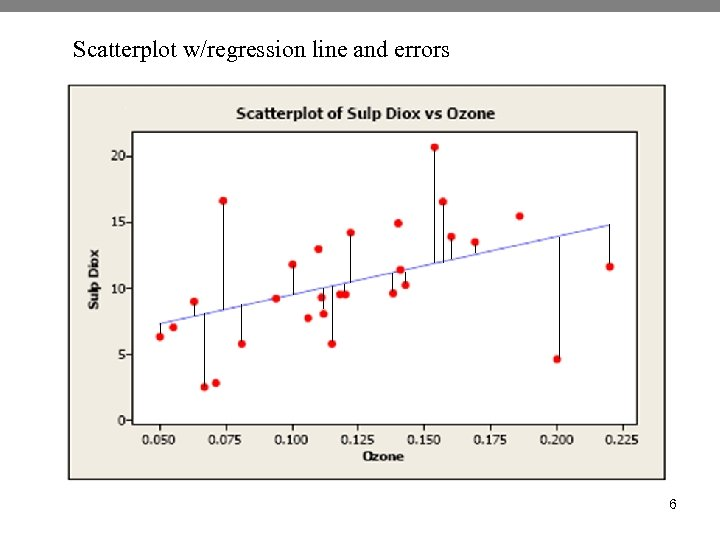Scatterplot w/regression line and errors 6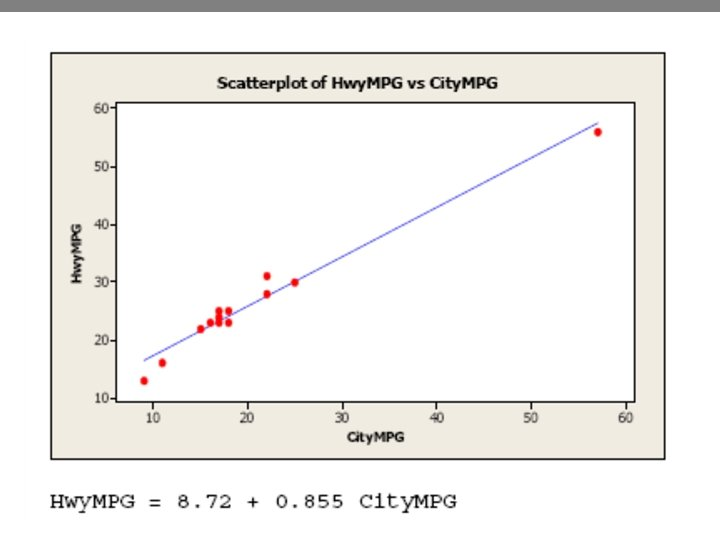7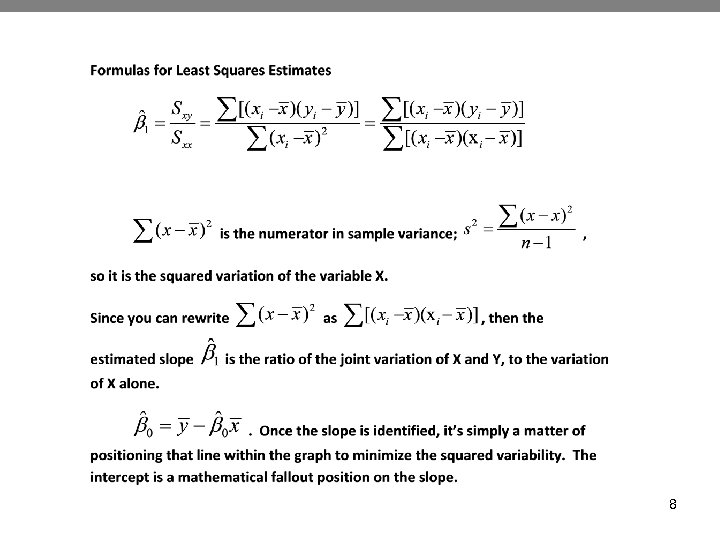8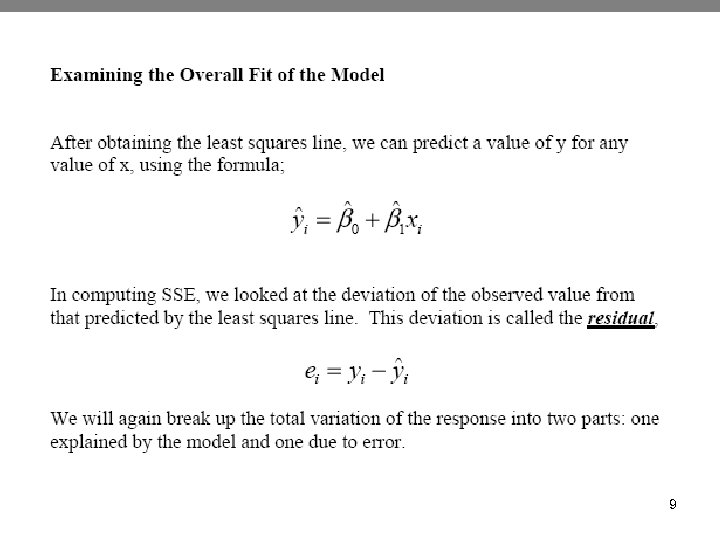9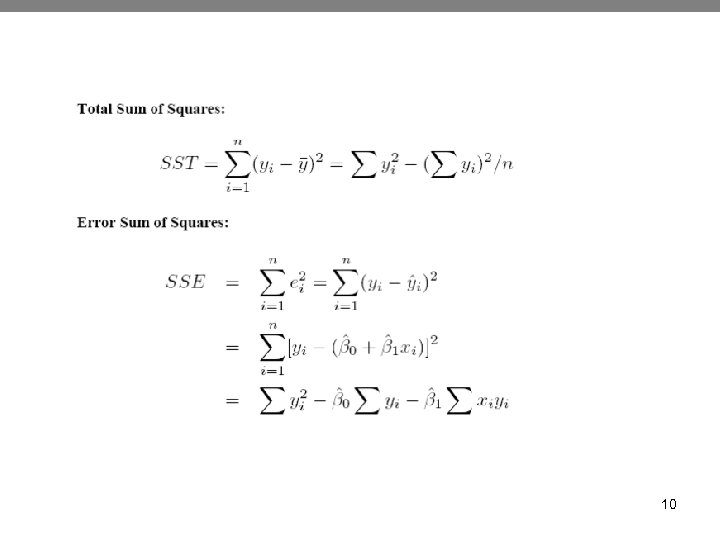10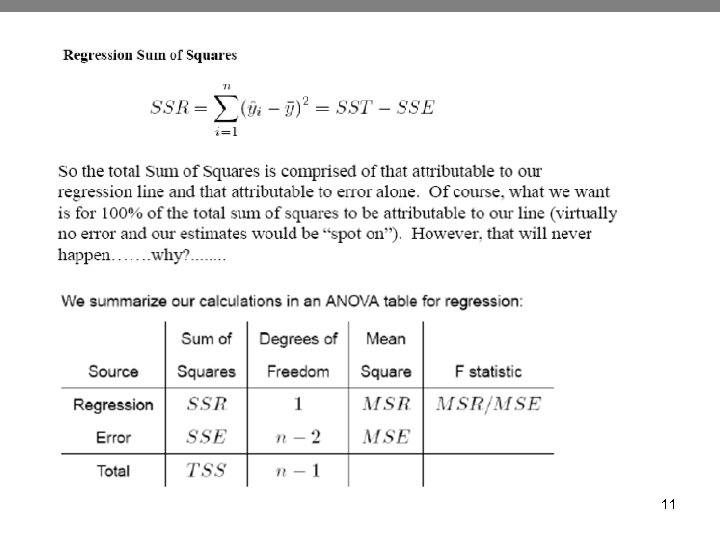11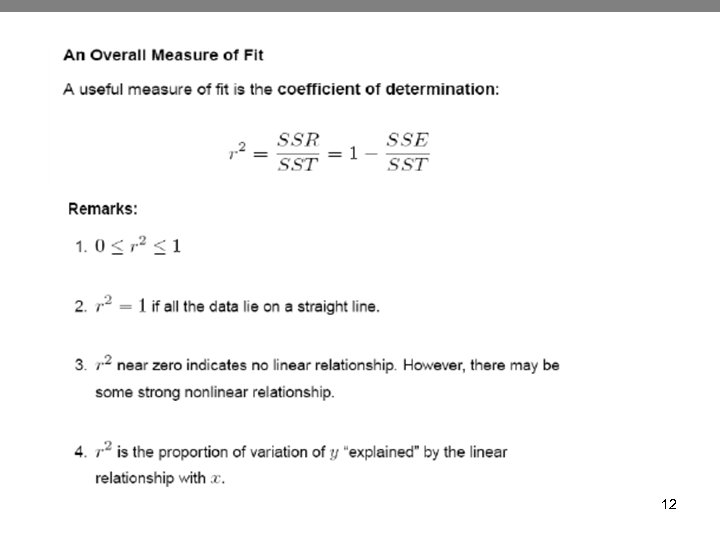12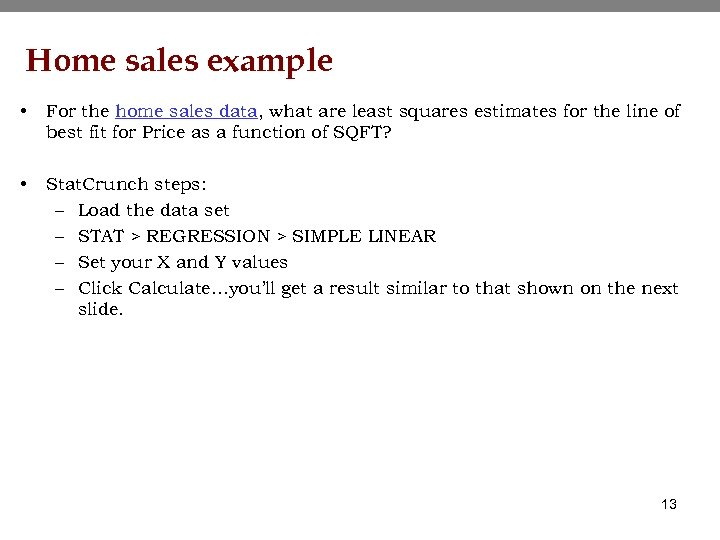Home sales example • For the home sales data, what are least squares estimates for the line of best fit for Price as a function of SQFT? • Stat. Crunch steps: – Load the data set – STAT > REGRESSION > SIMPLE LINEAR – Set your X and Y values – Click Calculate…you’ll get a result similar to that shown on the next slide. 13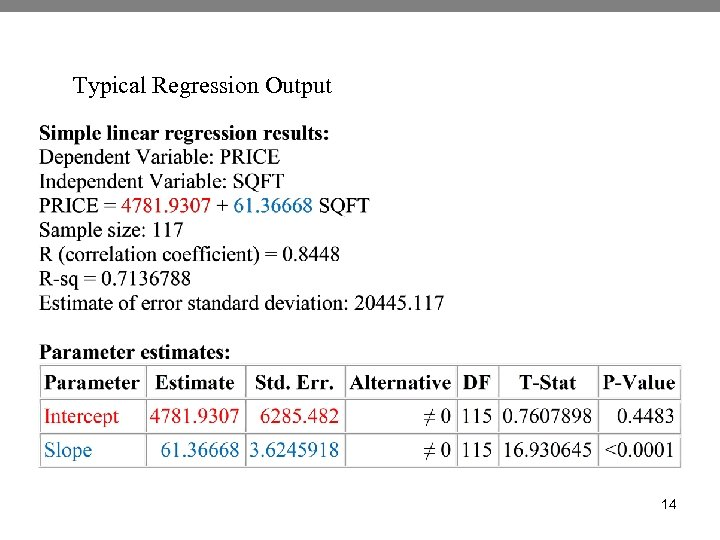Typical Regression Output 14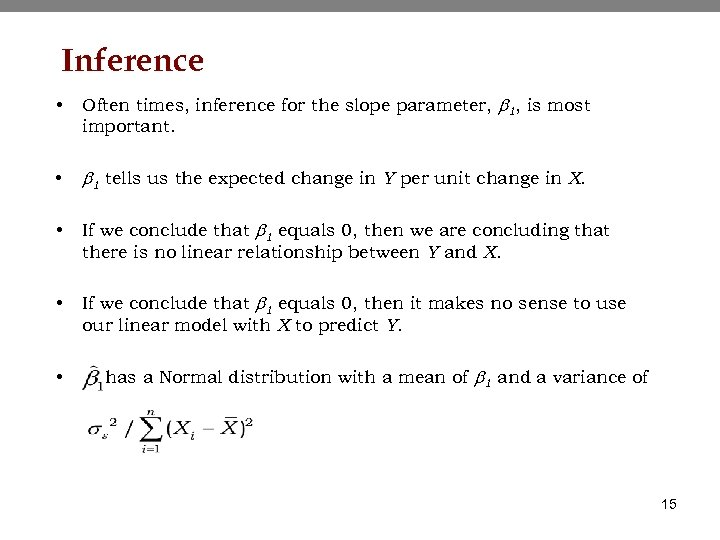Inference • Often times, inference for the slope parameter, b 1, is most important. • b 1 tells us the expected change in Y per unit change in X. • If we conclude that b 1 equals 0, then we are concluding that there is no linear relationship between Y and X. • If we conclude that b 1 equals 0, then it makes no sense to use our linear model with X to predict Y. • has a Normal distribution with a mean of b 1 and a variance of 15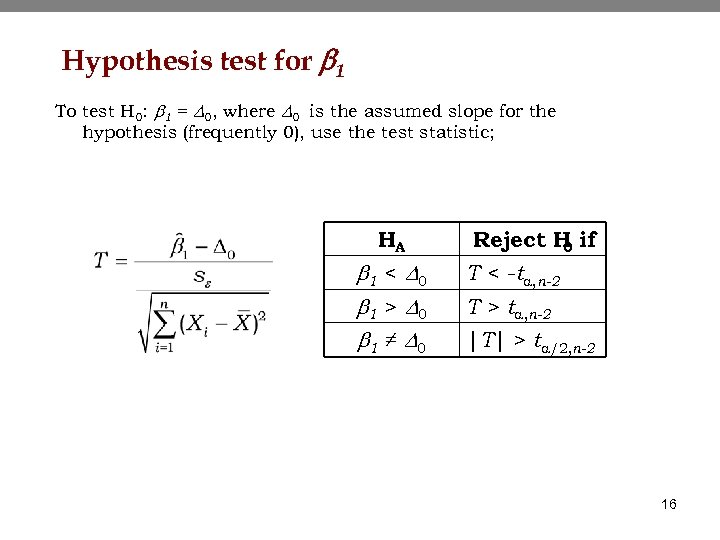Hypothesis test for b 1 To test H 0: b 1 = D 0, where D 0 is the assumed slope for the hypothesis (frequently 0), use the test statistic; HA b 1 < D 0 b 1 > D 0 b 1 ≠ D 0 Reject H if 0 T < -ta, n-2 T > ta, n-2 |T| > ta/2, n-2 16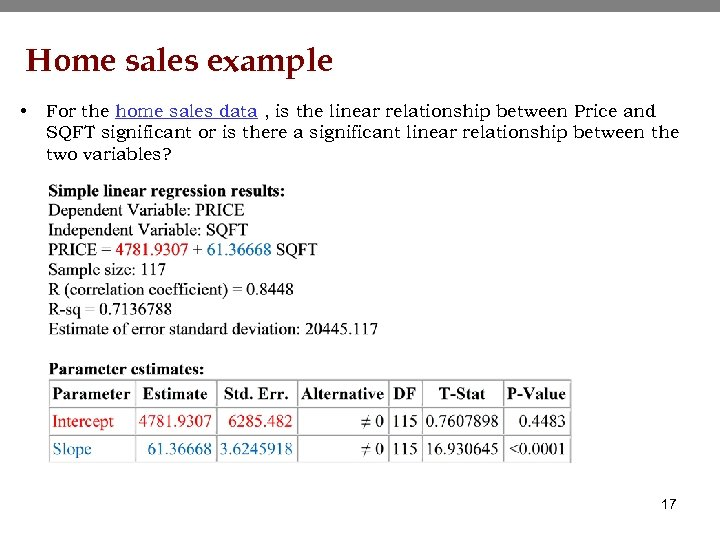Home sales example • For the home sales data , is the linear relationship between Price and SQFT significant or is there a significant linear relationship between the two variables? 17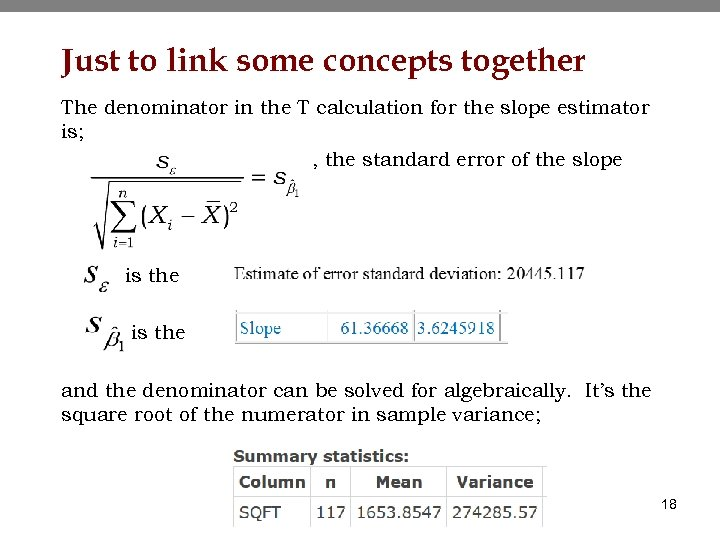Just to link some concepts together The denominator in the T calculation for the slope estimator is; , the standard error of the slope is the and the denominator can be solved for algebraically. It’s the square root of the numerator in sample variance; 18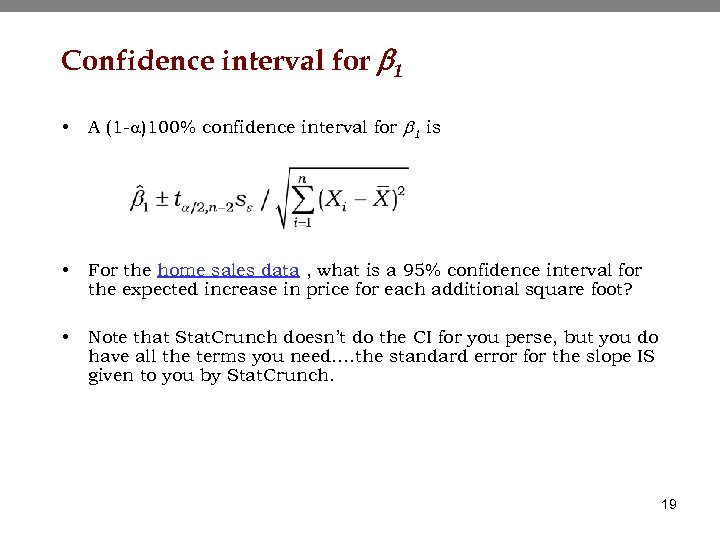Confidence interval for b 1 • A (1 -a)100% confidence interval for b 1 is • For the home sales data , what is a 95% confidence interval for the expected increase in price for each additional square foot? • Note that Stat. Crunch doesn’t do the CI for you perse, but you do have all the terms you need…. the standard error for the slope IS given to you by Stat. Crunch. 19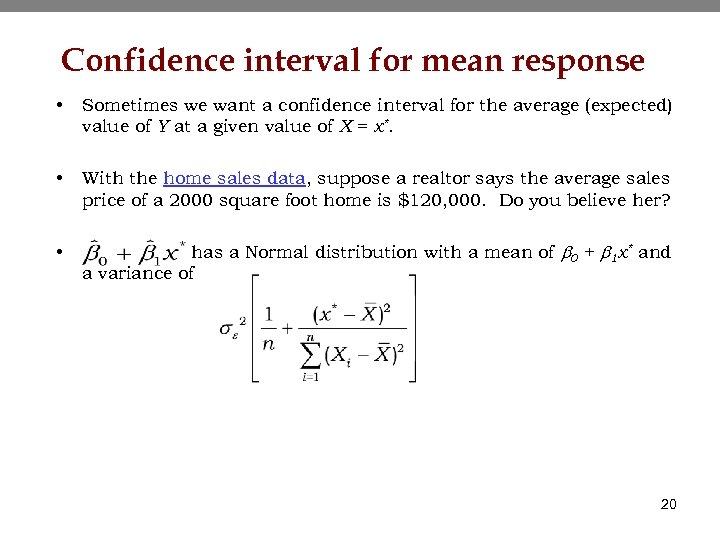Confidence interval for mean response • Sometimes we want a confidence interval for the average (expected) value of Y at a given value of X = x*. • With the home sales data, suppose a realtor says the average sales price of a 2000 square foot home is \$120, 000. Do you believe her? • has a Normal distribution with a mean of b 0 + b 1 x* and a variance of 20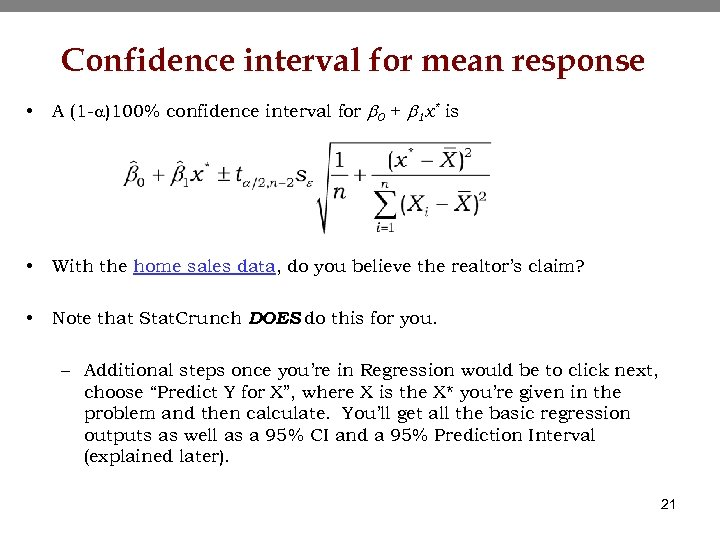Confidence interval for mean response • A (1 -a)100% confidence interval for b 0 + b 1 x* is • With the home sales data, do you believe the realtor’s claim? • Note that Stat. Crunch DOES do this for you. – Additional steps once you’re in Regression would be to click next, choose “Predict Y for X”, where X is the X* you’re given in the problem and then calculate. You’ll get all the basic regression outputs as well as a 95% CI and a 95% Prediction Interval (explained later). 21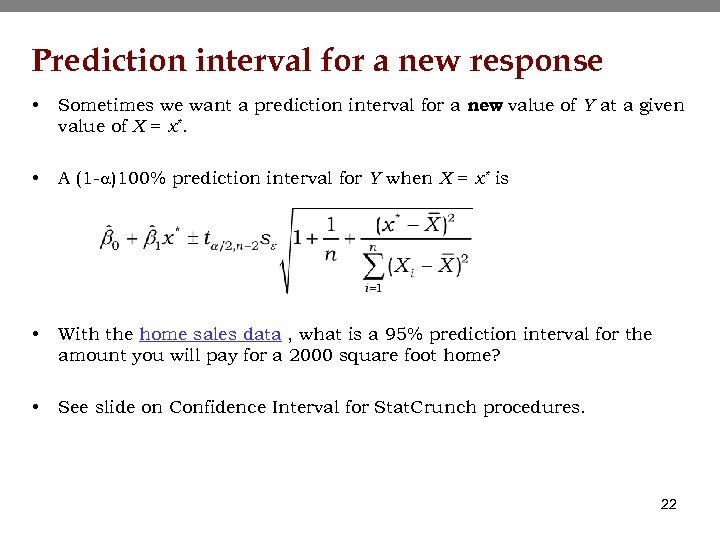Prediction interval for a new response • Sometimes we want a prediction interval for a new value of Y at a given value of X = x*. • A (1 -a)100% prediction interval for Y when X = x* is • With the home sales data , what is a 95% prediction interval for the amount you will pay for a 2000 square foot home? • See slide on Confidence Interval for Stat. Crunch procedures. 22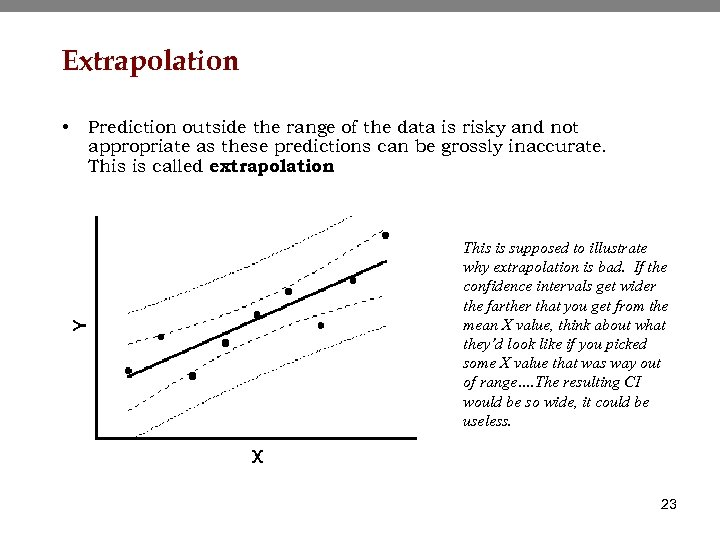Extrapolation • Prediction outside the range of the data is risky and not appropriate as these predictions can be grossly inaccurate. This is called extrapolation. This is supposed to illustrate why extrapolation is bad. If the confidence intervals get wider the farther that you get from the mean X value, think about what they’d look like if you picked some X value that was way out of range…. The resulting CI would be so wide, it could be useless. 23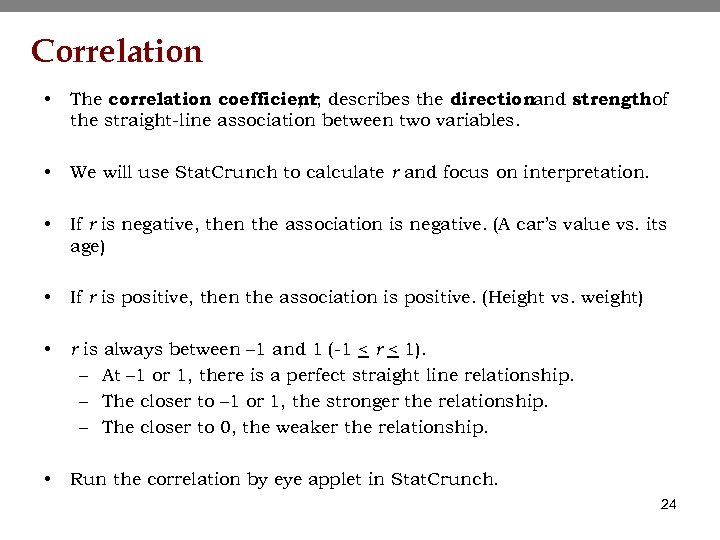Correlation • The correlation coefficient describes the directionand strengthof , r, the straight-line association between two variables. • We will use Stat. Crunch to calculate r and focus on interpretation. • If r is negative, then the association is negative. (A car’s value vs. its age) • If r is positive, then the association is positive. (Height vs. weight) • r is always between – 1 and 1 (-1 < r < 1). – At – 1 or 1, there is a perfect straight line relationship. – The closer to – 1 or 1, the stronger the relationship. – The closer to 0, the weaker the relationship. • Run the correlation by eye applet in Stat. Crunch. 24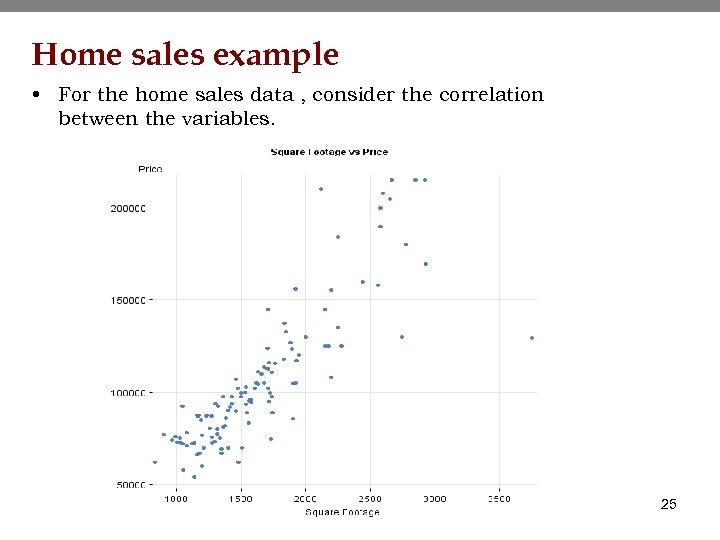Home sales example • For the home sales data , consider the correlation between the variables. 25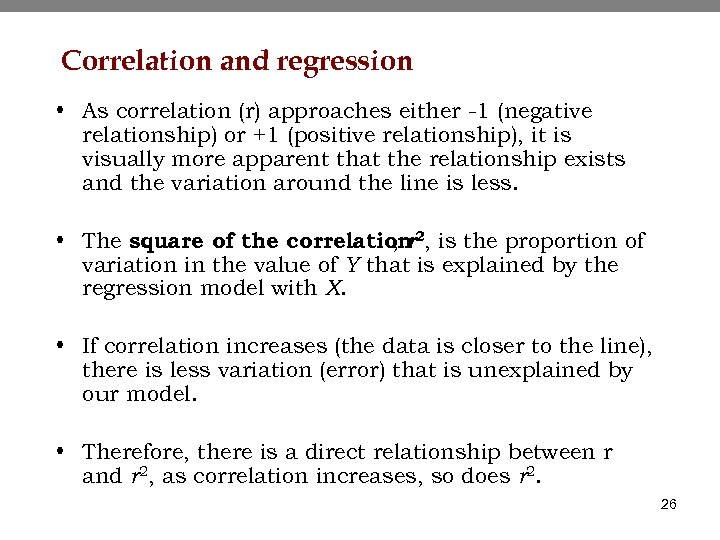Correlation and regression • As correlation (r) approaches either -1 (negative relationship) or +1 (positive relationship), it is visually more apparent that the relationship exists and the variation around the line is less. • The square of the correlation 2, is the proportion of , r variation in the value of Y that is explained by the regression model with X. • If correlation increases (the data is closer to the line), there is less variation (error) that is unexplained by our model. • Therefore, there is a direct relationship between r and r 2, as correlation increases, so does r 2. 26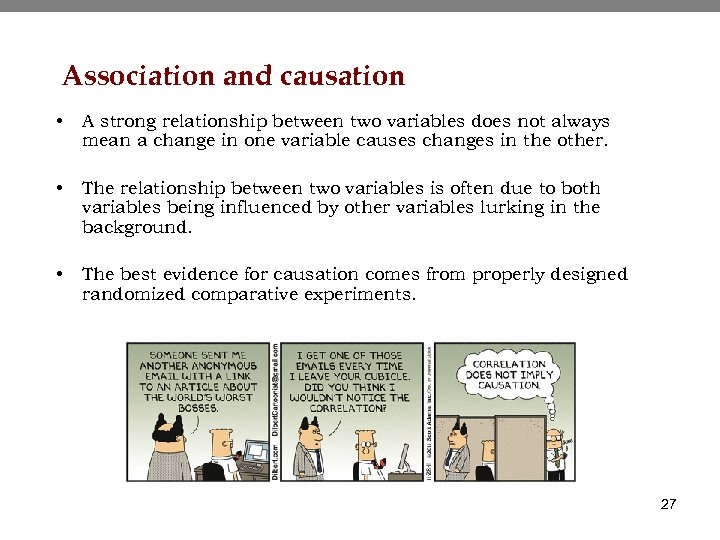Association and causation • A strong relationship between two variables does not always mean a change in one variable causes changes in the other. • The relationship between two variables is often due to both variables being influenced by other variables lurking in the background. • The best evidence for causation comes from properly designed randomized comparative experiments. 27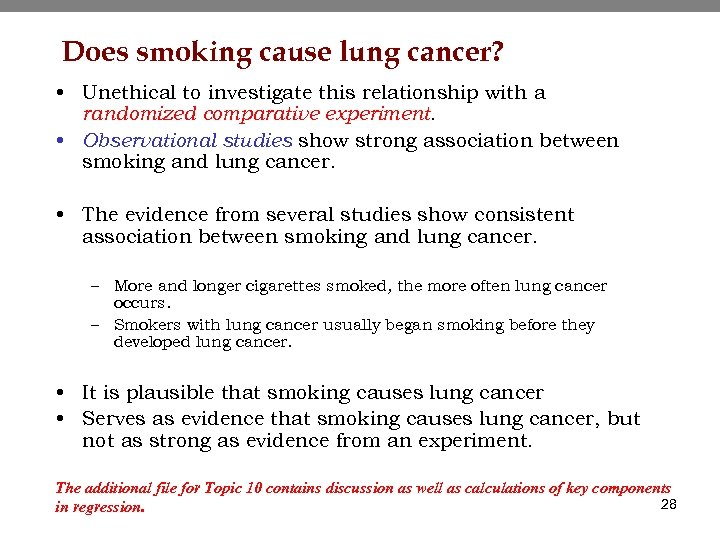Does smoking cause lung cancer? • Unethical to investigate this relationship with a randomized comparative experiment. • Observational studies show strong association between smoking and lung cancer. • The evidence from several studies show consistent association between smoking and lung cancer. – More and longer cigarettes smoked, the more often lung cancer occurs. – Smokers with lung cancer usually began smoking before they developed lung cancer. • It is plausible that smoking causes lung cancer • Serves as evidence that smoking causes lung cancer, but not as strong as evidence from an experiment. The additional file for Topic 10 contains discussion as well as calculations of key components 28 in regression.